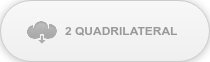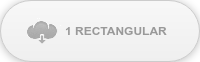# Expert Tips: Finite Element Method Basic 3#### Author: Seungwoo Lee, Ph.D., P.E., S.E.

##### Publish Date: 13 Dec, 2022

Continuing on to the third part of this multi-part blog, another option is a quadrilateral element. As always, let’s start with an example.Stress (ksi), One quadrilateral element case

The FEA shows deflection = 0.269 in. and stress = 40.00 ksi.

 Deflection (in) Stress (ksi) Beam theory 0.341 80 2 triangular elements 0.0249 1.804 128 triangular elements 0.306 66.85 1152 triangular element 0.350 86.55 2 high-order triangular element 0.285 50.73 32 high-order triangular element 0.355 81.94 1 quadrilateral element 0.269 40 2 quadrilateral element 0.333 60

Comparing the output from other elements, this quadrilateral element (2 dofs/node × 2 nodes = 4 dofs) gives much better results than those from linear triangular elements (2 dofs/node × 2 nodes = 4 dofs) but close to those from high-order triangular elements (2 dofs/node × 6 nodes = 12 dofs). As can be seen, this quadrilateral element is a very economical tool, at least for this example.

For linear triangular elements, the shape function is as follows (or the displacement in an element is defined as follows).

u or v= a1+a2x+a3y

This is a linear function and has no missing terms. There are three nodes and three unknowns, so we can solve this equation.

For high-order triangular elements, the shape function is

u or v= a1+a2x+a3y+a4x2+a5xy+a6y2

This is a quadratic function and has no missing terms. There are six nodes and six unknowns, so we can solve this equation.

For quadrilateral elements, we have four nodes, so we cannot use a linear function (this is an indeterminate case). We cannot use a (complete) quadratic function because this is an impossible case. We can not find six unknowns, only with four given values.

One of the solutions is to omit two terms in the (complete) quadratic function, and our brilliant fore-engineers selected the following shape function.

u or v= a1+a2x+a3y+a4xy

As can be seen, this is an “incomplete” quadratic function called “bilinear.”

Why not select something like the following shape function?

u or v= a1+a2x2+a3xy+4y2

That is because we must include constant and linear terms to consider rigid-body motion and constant strain conditions. Also, the shape function shall be “balanced,” and we cannot use something like the followings.

u or v= a1+a2x+a3y+a4x2

We can get the strain as the derivative of the displacement function,

x=∂u∂x=a2+a4y

The x-direction strain εx is constant in the x-direction and only varies along the y-direction. In other words, the x-direction stress σx is constant along the x-direction within an element. This is the reason why we get constant stress at the element top along the x-direction.

Some smart engineers can guess the value 40 ksi is the average between the left end and right end, and the stress at the right end top is zero, so the correct value at the left end top is close to 80 ksi without further calculation. Also, they can guess that we do not need more elements in the vertical direction to get the refined value of σx, we only need to divide into more elements along the x-direction.

What happens if we use two quadrilateral elements in the transverse direction?

We can guess σx may be close to (80 ksi + 40 ksi)/2 = 60 ksi with two elements, and the FEA result confirms this assumption as below.Stress (ksi), Two quadrilateral element case

As expected, we can get more exact values if the loading case is pure bending.

Pure bending case

 Deflection (in) Stress (ksi) Beam theory 0.032 5 2 high-order triangular element 0.0315 5.216 1 quadrilateral element 0.032 5Stress (ksi), One quadrilateral element case, pure bending case